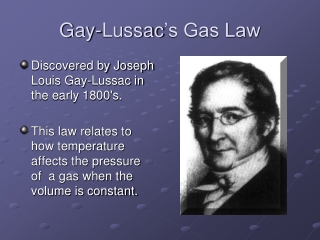DownloadDownload PresentationGay-Lussac ’s Gas Law

# Gay-Lussac ’s Gas Law

Télécharger la présentation## Gay-Lussac ’s Gas Law

- - - - - - - - - - - - - - - - - - - - - - - - - - - E N D - - - - - - - - - - - - - - - - - - - - - - - - - - -
##### Presentation Transcript

1. Gay-Lussac’s Gas Law • Discovered by Joseph Louis Gay-Lussac in the early 1800's. • This law relates to how temperature affects the pressure of a gas when the volume is constant.

2. Temperature vs. Pressure • Lussac’s Gas Law states that if the temperature of a gas is increased, and the volume is held constant, the pressure of the gas will also increase. • On the other hand if the temperature of a gas is decreased, and the volume is held constant, the pressure of the gas will also decrease.

3. Temperature vs. Pressure • When the temperature is increased the gas molecules will move faster and they will impact the container walls more often. This increases the gas pressure inside the rigid container (volume stays constant).

4. Direct Relationship • Gay-Lussac's Law is a direct mathematical relationship. This means that there are two connected values and when one goes up, the other also increases.

5. P ÷ T = k • The mathematical form of Gay-Lussac's Law is: P ÷ T = k • This means that the pressure-temperature fraction will always be the same value if the volume and amount of gas remain constant.

6. As with the other laws, the exact value of k is unimportant in our context. • It is important to know that the PT data pairs obey a constant relationship, but it is not important to know the exact value of this constant. • Besides which, the value of k would shift based on what pressure units (atm, mmHg, or kPa) you were using.

7. We know this: P1 ÷ T1 = k • And we know this: P2 ÷ T2 = k • Since k = k, we can conclude that: P1 ÷ T1 = P2 ÷ T2

8. Problem # 1 • 10.0 L of a gas is found to exert 97.0 kPa at 25.0°C. What would be the required temperature (in Celsius) to change the pressure to standard pressure?

9. Solution STEP 1: Change 25.0°C to 298.0 K and remember that standard pressure in kPa is 101.325. STEP 2: Insert values into the equation. STEP 3: Solve for x

10. Final Answer • The answer is 311.3 K, but the question asks for Celsius, so you subtract 273 to get the final answer of 38.3°C.